# Solenoid Driver Circuit

Published  July 23, 2018   4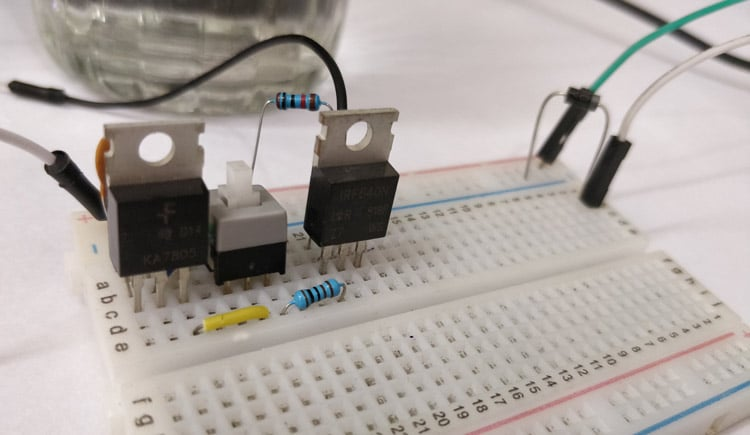Solenoids are very commonly used actuators in many process automation systems. There are many types of solenoid, for instance there are solenoid valves which can be used to open or close water or gas pipe lines and there are solenoid plungers which are used to produce linear motion. One very common application of solenoid that most of us would have come across is the ding-dong door bell. The Door bell has a plunger type solenoid coil inside it, which when energised by AC power source will move a small rod up and down. This rod will hit the metal plates placed on either side of the solenoid to produce the soothing ding dong sound.

Although there are many types of solenoid mechanisms available, the most basic thing remains the same. That is, it has a coil wound over a metal (conductive) material. When the coil is energised this conductive material is subjected to some mechanical movement which is then reversed through a spring or other mechanism when de-energised. Since the solenoid involves coil they often consume a large amount of current making it mandatory to have some type of driver circuit to operate it. In this tutorial we will learn how to build driver circuit to control a Solenoid valve.

### Materials Required

• Solenoid Valve
• 7805 Regulator IC
• IRF540N MOSFET
• Diode IN4007
• 0.1uf Capacious
• 1k and 10k Resistors
• Connecting wires

### What is a Solenoid and how does it work?

A solenoid is a device that converts electrical energy into mechanical energy. It has a coil wound over a conductive material, this set-up acts as an electromagnet. The advantage of an electromagnet over natural magnet is that it can be turned on or off when required by energising the coil. Thus when the coil is energised then according to faradays law the current carrying conductor has a magnetic field around it, since the conductor is a coil the magnetic field is strong enough to magnetise the material and create a linear motion.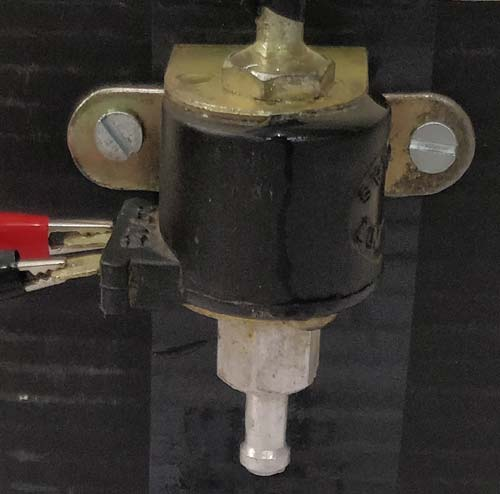During this process the coil draws a large amount of current and also produces hysteresis problem, hence it is not possible to drive a Solenoid coil directly though a logic circuit. Here we are using a 12V solenoid valve which is commonly used in controlling the flow of liquids. The solenoid draws a continuous current of 700mA when energised and a peak of nearly 1.2A so we have to consider these things while designing the driver circuit for this particular Solenoid valve.

### Circuit Diagram

The complete circuit diagram for Solenoid driver circuit is shown in the image below. We will understand why it is designed so, once after taking a look at the complete circuit.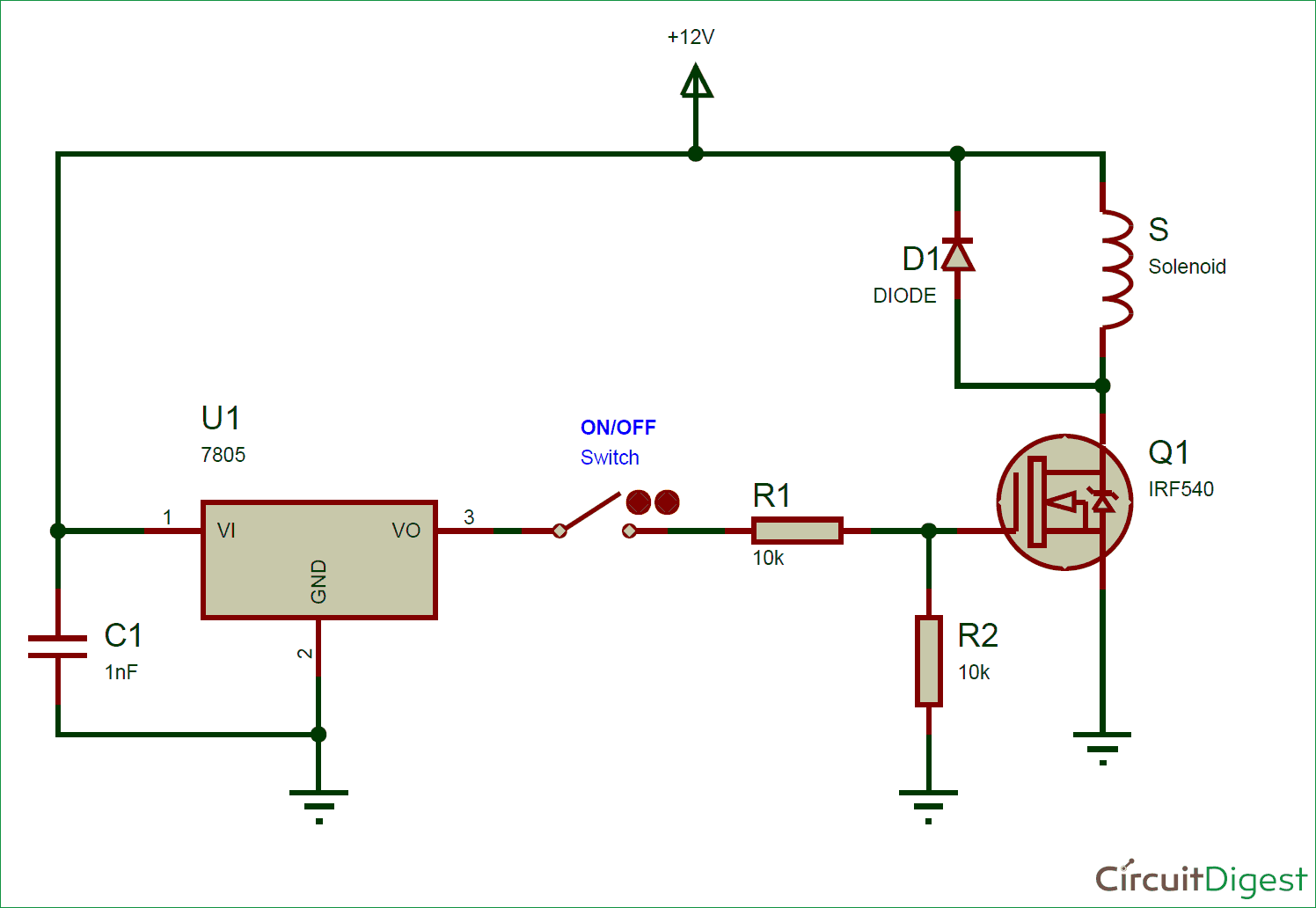As you can see the circuit is very simple and easy to build, hence we can test this using a small breadboard connection. A solenoid can simple be turned on by powering 12V across its terminals and turned off by powering it off. To control this turn on and off process using a digital circuit we need a switching device like the MOSFET and thus it is the important component in this circuit. The following are the parameters that you have to check while selecting the MOSFET.

Gate Source Threshold Voltage VGS(th): This is the voltage that has to be supplied to MOSFET to turn it ON. Here the threshold voltage value is 4V and we are supplying a voltage of 5V which is more than enough to turn on the MOSFET completely

Continuous Drain current:  The continuous drain current is the maximum current that can be allowed to flow through a circuit. Here our solenoid consumes a maximum peak current of 1.2A and the rating of our MOSFET is 10A at 5V Vgs. So we are more than safe with the current rating of the MOSFET. It is always recommended to have some upper marginal difference between the actual value and rated value of current.

Drain-Source On-State Resistance:  When the MOSFET is fully turned on it has some resistance between the Drain and Source pin, this resistance is called as on state resistance. The value of this should be as low as possible else there will be huge voltage drop (ohms law) across the pins resulting in not sufficient voltage for the Solenoid to turn on. The value of on-state resistance here is only 0.077Ω.

You can look at the datasheet of your MOSFET if you are designing the circuit for some other Solenoid application.  A 7805 Linear Regulator IC is used to convert the 12V input supply to 5V, this voltage is then given to the Gate pin of the MOSFET when the switch is pressed though a 1K current limiting resistor. When the switch is not pressed the gate pin is pulled down to ground through a 10k Resistor. This keeps the MOSFET turned off when the switch is not pressed. Finally a diode is added in anti-parallel direction to prevent the solenoid coil from discharging into the power circuit.

### Working of Solenoid Driver Circuit

Now that we have understood how the Driver circuit works lets test the circuit by building it on a bread board. I have used a 12V adapter for powers supply and my hardware setup looks something like this when completed.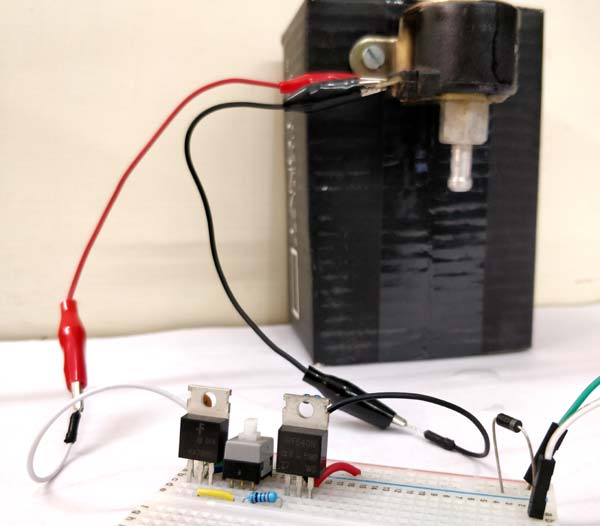When the switch in between is pressed the +5V supply is provided to the MOSFET and it turns on the Solenoid. When the switch is pressed again it disconnects the +5V supply to MOSFET and the solenoid goes back to off state. The turning on and off the solenoid can be noticed by the clicking sound made by it, but to make it a bit more interesting I have connected the solenoid valve to a water pipe. By default when solenoid is off the value is closed and hence no water comes out through other end. Then when the solenoid is turned on the value gets open and water flows out. The working can be visualised in the video below.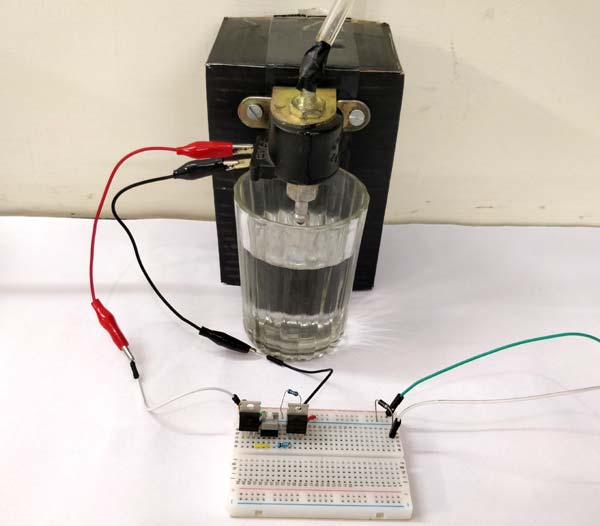Hope you understood the project and enjoyed building it, if you had faced any problem then feel free to post them in the comment section or use the forum for technical help.

Video

Submitted by Chris Arena on Mon, 07/23/2018 - 19:47

### need for 7805?

What's the purpose of the +5 V regulator? It's an added 2 parts that might not be necessary.
The voltage applied to the gate will be about 2.5V with the resistive divider you've set up, which is a bit short of 4V, but 4V is the max Tgs.
It might be a better example to do that circuit with the intention of driving it from an Arduino or Pi, which many would be using I expect.
Chris

### The mosfet will not turn off

The mosfet will not turn off if the gate pin is not pulled down to ground

### resistor values

The parts list states 2 resistors, one as 1k the other as 10k

The diagrahm shows both as 10k

The write up says the 10k is to ground the mosfet

So I assume the other is actually 1k and goes to the regulator?

Thanks for doing this for other than Arduino as I am using this with a physical switch.

How hot does the 7805 get?  Will it require a heat sink?

Thanks!!!!

### The schematic shows the gate…

The schematic shows the gate driven from the middle of a voltage divider that divides the 5 volts from the regulator by two, resulting in 2.5V.

But the text says:

"Here the threshold voltage value is 4V and we are supplying a voltage of 5V which is more than enough to turn on the MOSFET completely."

If we assume that R1 was supposed to be 1K, the we're feeding the gate 10k/11k * 5V which is 4.5V.  That's enough to turn on the MOSFET, but it's not 5V.